# Understanding contravariance

In this lesson we learn about the concept of contravariance, contravariant functors, and the `Contravariant` typeclass defined in the `contravariance` package.`contravariant` on Hackage. Note that as of GHC 8.6.1 release, `Data.Functor.Contravariant` is included in `base`! The remaining modules from the `contravariant` package are not in `base`.

A functor in Haskell is a type constructor along with a lawful implementation of the function `fmap`, the primary method of the `Functor` typeclass. When given a function, `z0 -> z1`, and an `f z0` as inputs, `fmap` returns an `f z1`. The `f` in these types is the type constructor.

``````class Functor (f :: * -> *) where
fmap :: (z0 -> z1) -> f z0 -> f z1``````

This is often referred to as “lifting”, a metaphor for taking something (in this case, a function) from one context to another. We can write the type of `fmap` with extra parentheses to make the “lifting”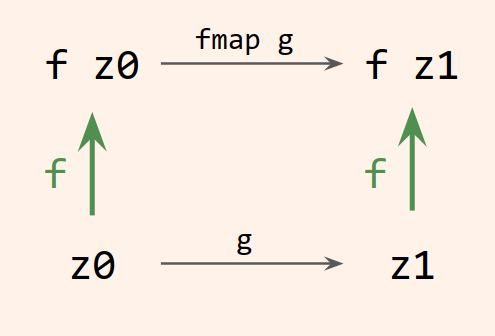more clear:

``fmap :: (z0 -> z1) -> (f z0 -> f z1)``

The function `z0 -> z1` is transformed into a function `f z0 -> f z1`, and we say that the function is lifted into `f`.

These are covariant functors, although in general we do not talk about them that way until we’ve understood there are also functors with flipped around arguments called contravariant functors.

## There are more functors in heaven and Earth

The `Functor` typeclass provides one basic way to lift, but lifting doesn’t stop there; several other typeclasses provide lifting operations. `Applicative`, `Monad`, and `Bifunctor` can be viewed as `fmap` with a little something extra. These functors all have lawful implementations of `fmap`, but not all functors have lawful implementations of the lifting operations from these other classes. There are more functors than there are applicatives; more applicatives than there are monads.

`Contravariant` and `Profunctor`, though, are quite different. We’ll just be talking about `Contravariant` here. After this, you may wish to go on to `Bifunctor` and `Profunctor`. Very many functors are covariant functors, while relatively few functors are contravariant.

When you start exploring contravariant functors, you may at first think the type of the primary method, `contramap`, is contraintuitive: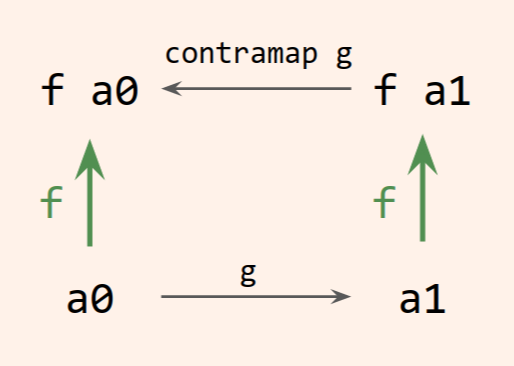``````class Contravariant (f :: * -> *) where
contramap :: (a0 -> a1) -> f a1 -> f a0``````

Compared to `fmap`, this seems strange – how do we pass an `a0` to our `a0 -> a1` function when what we have is an `f a1`? How can we transform an `a0 -> a1` into an `f a1 -> f a0`? Somehow a result type will need to be adapted into an input type.

The first thing to do in trying to understand `Contravariant` is to understand what sorts of type constructors are contravariant. Let’s ask the REPL using the `:info` command: Some output from GHCi has been elided here for brevity.

``````λ> import Data.Functor.Contravariant

λ> :info Contravariant
class Contravariant (f :: * -> *) where
contramap :: (a0 -> a1) -> f a1 -> f a0
instance Contravariant Predicate
instance Contravariant (Op z)
instance Contravariant Equivalence
instance Contravariant Comparison

λ> :info Predicate
newtype Predicate a = Predicate {getPredicate :: a -> Bool}

λ> :info Op
newtype Op z a = Op {getOp :: a -> z}

λ> :info Equivalence
newtype Equivalence a
= Equivalence {getEquivalence :: a -> a -> Bool}

λ> :info Comparison
newtype Comparison a
= Comparison {getComparison :: a -> a -> Ordering}``````

Notice what these types all share: They are all wrappers around functions. That narrows the field of what we need to understand considerably!

That’s especially interesting because there is also a covariant functor related to functions; that is, there is a lawful `Functor` instance based on the type constructor `(->)`. Let’s back up for a moment and see what the covariant functor can teach us.

## A `Functor` of functions

When you first learn about the `Functor` class with its definition of `fmap`, you may have thought of those `f` types as “containers” For an argument that functors really are containers, see Functors are Containers by Bartosz Milewski. or “wrappers” For a more lighthearted take, try Monads are like burritos by Mark Dominus. and learned to understand them first as things like `Maybe` and lists. That understanding works fine until you find out about the functor of functions, when suddenly the `f` is `(a ->)` and the world shifts just a bit.

Typically when learning Haskell, people learn about function composition before they learn about the `Functor` typeclass, or perhaps they’ve learned about composition from a math class prior to ever learning Haskell. At any rate, it is often a surprise for people to learn that function composition is the `fmap` of functions, or, more precisely for the type constructor `((->) a)`. Let’s take a moment to understand why this is so.

As we said above, functors lift a function `z0 -> z1` to a function `f z0 -> f z1` where `f` is some type constructor. The type constructor for functions looks like this (simplified a bit):

``data (->) a z``

It’s a special datatype, and yet for the purposes of talking about the `Functor` instance, it works similarly to a pair or two-tuple type. As usual for type constructors with more than one type parameter, we must partially apply this constructor to write the instance.

``````instance Functor ((->) a) where
fmap = _``````

Note that `(->) a` is equivalent to `(a ->)`.

We’ve already said that this implementation of `fmap` is the same as `(.)`, but we want to prove it to ourselves.

Recall the general type of `fmap`:

``fmap :: (z0 -> z1) -> f z0 -> f z1``

We know the `f` here will be `(->) a`, so `f z0` is `((->) a) z0` and `f z1` is `((->) a) z1`. Thus our `fmap` specialized to `(->) a` looks like this: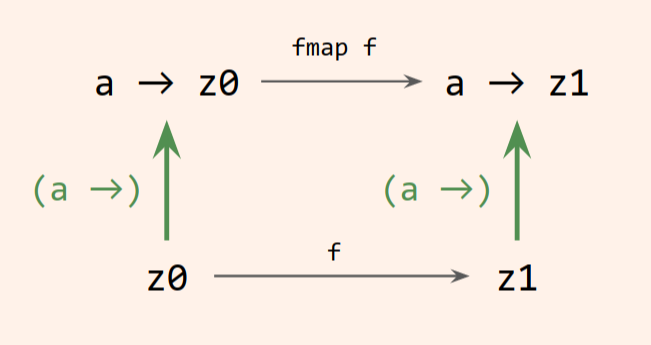``(z0 -> z1) -> ((->) a) z0 -> ((->) a) z1``

We can rewrite that with the arrows in infix notation as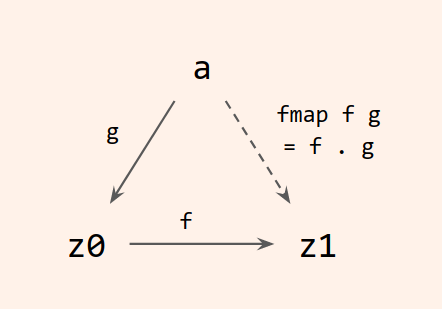``(z0 -> z1) -> (a -> z0) -> (a -> z1)``

Let’s compare that directly to the type of function composition:

``(.) :: (b -> c) -> (a -> b) -> (a -> c)``

If we label the `(->) a` type constructor `f`, then we see how we get directly from the type of composition to the type of `fmap`.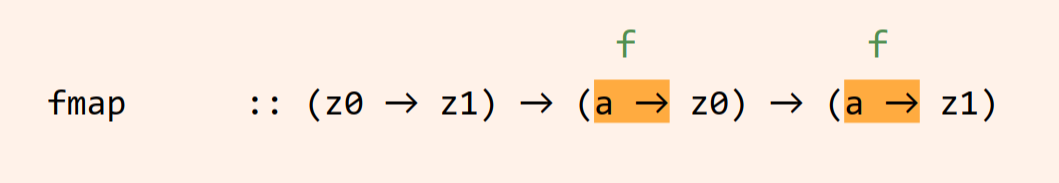Thus we can finish our `fmap` implementation by defining it as function composition.

``````instance Functor ((->) a) where
fmap :: (z0 -> z1) -> (a -> z0) -> (a -> z1)
fmap = (.)``````

We can think of this as lifting a function into a context of another function. Lifting a function into a function context provides a context in which it can accept as input the output of another function. If we have a function `f :: a -> z0` but we need a function `a -> z1`, then a function `g :: z0 -> z1` takes us there via `fmap g`. When we lift `g` into the context of `(a ->)` via `fmap g`, we adapt `f` from `a -> z0` to `a -> z1` by post-processing its result.

To put another way: The type constructor `(->) a z` is covariant in its second argument.

## Contravariant composition

Now it’s time for something completely different.

Contravariant functors reverse the direction of composition.

This gives us a good place to begin our investigation into contravariance – by writing a reversed function composition.

We want to write a “contra-composition” function that compares to regular (covariant) composition like this:

``````(.)        :: (b -> c) -> (a -> b) -> a -> c
contraComp :: (a -> b) -> (b -> c) -> a -> c``````

The implementations also resemble each other.

``````(.)        f g x = f (g x)
contraComp f g x = g (f x)``````

We can see that the only difference here is that it we have flipped the order of the arguments. And if we experiment with these in the REPL, we would get the expected results:

``````λ> comp (< 10) length [1..20]
False

λ> contraComp length (< 10) [1..20]
False``````

This isn’t a super compelling example; why would you particularly want to flip the application order around like this? We’ll ask you to defer judgment on that question until we can get to some more interesting examples in a bit.

But the upshot is:

1. If we have a function `a -> z0` and we need `a -> z1` then we need to change our result types, so we need an “adaptor” function `z0 -> z1` to get there.
2. If we have a function `a0 -> z` and we need `a1 -> z`, we have to change the input type. We need a function from `a1 -> a0` to get there.

With covariant composition, we wanted to change the result type and did so by passing it to an adaptor function. Now we want to change the input type by pre-processing the input before passing it off to our original function.

Contrasting `Functor` versus `Contravariant`, we can see this by annotating the composition types like this: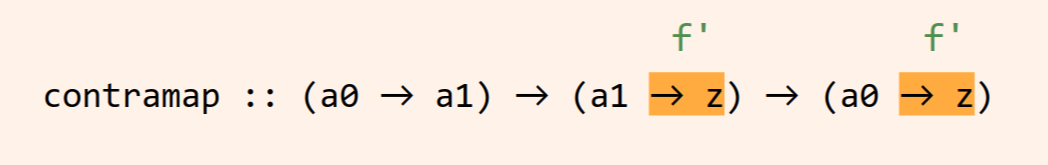With covariant right-to-left function composition, the `f` constructor represents fixing the type of the function’s input. With the contravariant, left-to-right functor of functions, the `f'` constructor represents fixing the type of the function’s result.

By “fixing”, we mean a shorthand for it being a free, or unbound, variable. For example, if we say, “consider `Either a b` where `a` is fixed”, we mean “consider `\b -> Either a b`.” The `a` is arbitrary, but for the purposes of the discussion it’s not the input variable for the functor – `b` is the input variable for the functor. So when we say that `(->) a b` is covariant in its second argument and fixes the type of the input, we’re saying that, whatever the type of `a`, it isn’t the object of our concern; we’re not going to touch it or transform it or adapt it. It’s a black box as far as our composition is concerned.

## On to `Contravariant`

What we just did above is a good gateway to understanding the `Contravariant` class and its `contramap` method, because `contramap` is exactly the flipped composition that we just wrote!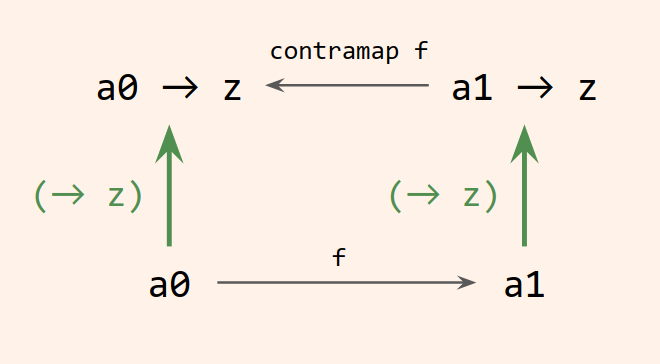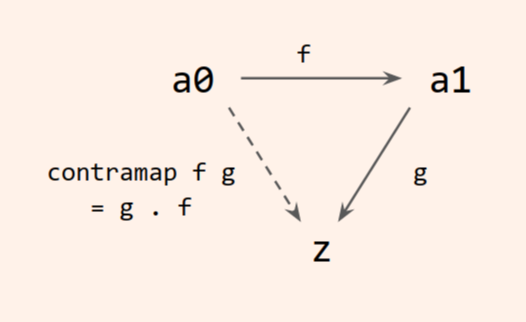``````instance Functor (a ->) where
fmap = (.)

instance Contravariant (-> z) where
contramap = flip (.)``````

That last instance doesn’t actually compile, unfortunately. We need to wrap the function type in a newtype with the type arguments ordered so that we can fix `z` and write the `Contravariant` instance with `a` as the bound type variable.

We have various choices for newtypes, but the most basic is `Op.` `Op` is a wrapper around a function with its arguments flipped:

``newtype Op z a = Op { getOp :: a -> z }``

Let’s talk about this declaration for a moment. `Op` has two parameters; when we look at the data constructor, we see that the first parameter is the result of a function, and the second parameter is the input. The `getOp` function allows us to conveniently unwrap an `Op` value into this underlying function: given an `Op z a` value as its first argument, it gives us a function `a -> z`.

## `Op`

Let’s rewrite our `contraComp` example from above, but using `Op`.

``````newtype Op z a =
Op { getOp :: a -> z }``````

The `contramap` definition for `Op` should look familiar, with a bit of extra plumbing:

``````instance Contravariant (Op a) where
contramap f g = Op (getOp g . f)``````

Compare this to our previous definition of flipped function composition, `contraComp`:

``contraComp f g x = g (f x)``

Or, equivalently:

``contraComp f g = (g . f)``

Let’s see if we can turn our completely contrived `length` example from above into a fancy `contramap` example. We want to check lists to see if they are of length 10:

``````isTen :: Op Bool Int
isTen = Op (== 10)

isListOfTen :: Op Bool [a]
isListOfTen = contramap length isTen``````

Notice that because `Op` has its parameters in the reverse order of the wrapped function, the `Bool` results are the first type argument, while the inputs are the second type argument. `isListOfTen` takes a list as its input, while `isTen` takes an `Int` as input; each returns a `Bool`.

Remember that `contramap length isTen` is equivalent to `(getOp isTen) . length`.

In the REPL:

``````λ> getOp isListOfTen [1..100]
False

λ> getOp isListOfTen [1..10]
True``````

## `Predicate`

What we just wrote using `Op Bool` is perhaps better captured by the newtype `Predicate`, which is a wrapper around `a -> Bool` functions.

``````newtype Predicate a =
Predicate { getPredicate :: a -> Bool }``````

Let’s refactor to use `Predicate` and its `contramap`:

``````isTen :: Predicate Int
isTen = Predicate (== 10)

isListOfTen :: Predicate [a]
isListOfTen = contramap length isTen``````

Use `getPredicate` to apply this to a list and run it:

``````λ> getPredicate isListOfTen [1..100]
False

λ> getPredicate isListOfTen [1..10]
True``````

Let’s add an additional function to filter a list of lists with our predicate:

``````filterLists :: [[a]] -> [[a]]
filterLists = filter (getPredicate listLength)``````

## `Equivalence`

Another contravariant functor worth looking at is `Equivalence`. `Equivalence` is a newtype wrapper around equality relations.

``````newtype Equivalence a =
Equivalence { getEquivalence :: a -> a -> Bool }``````

The `contravariant` package also provides a function called `defaultEquivalence` that puts `==` into the `Equivalence` context, much the way our `isTen` functions above put `(== 10)` into the `Op` or `Predicate` context.

``````defaultEquivalence :: Eq x => Equivalence x
defaultEquivalence = Equivalence (==)``````
``````λ> getEquivalence defaultEquivalence 4 5
False

λ> getEquivalence defaultEquivalence 4 4
True``````

Now we can use this to check for equivalence on only one side of a tuple by contramapping

``````fstEquivalence :: Eq a => Equivalence (a, b)
fstEquivalence =
contramap fst defaultEquivalence

sndEquivalence :: Eq b => Equivalence (a, b)
sndEquivalence =
contramap snd defaultEquivalence``````

If we compare two tuples using a default equivalence, it checks both elements for equality:

``````λ> getEquivalence defaultEquivalence (1, 2) (1, 3)
False

λ> getEquivalence defaultEquivalence (1, 2) (1, 2)
True``````

But using the equivalences we wrote above, we can also compare them on just one side or the other:

``````λ> getEquivalence fstEquivalence (1, 2) (1, 3)
True

λ> getEquivalence sndEquivalence (1, 2) (1, 3)
False``````

In summary, for covariant functors, the unlifted and lifted functions point in the same direction, `z0 -> z1`. With contravariance, the unlifted and lifted functions point in opposite directions: one goes `a0 -> a1`, the other goes `a1 -> a0`, but reversing the order of composition lets us get where we need to go.

## What’s next

While you can imagine functions like this, that filter some inputs by one or more predicates, all of this could have been written another way. You may have even invented flipped function composition on your own without realizing it had a name! But contravariance really starts to shine in the context of bifunctors. Bifunctors that are contravariant in their first argument and covariant in their second argument are called profunctors.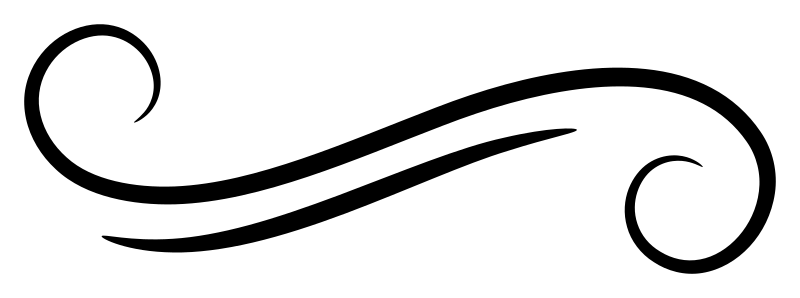Join Type Classes for courses and projects to get you started and make you an expert in FP with Haskell.